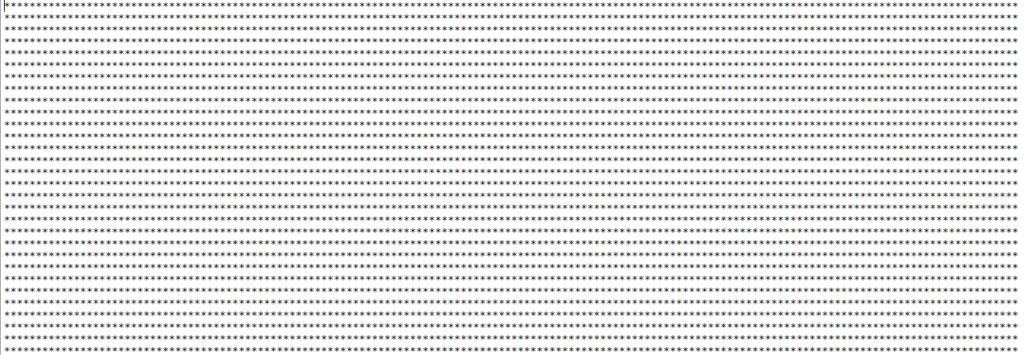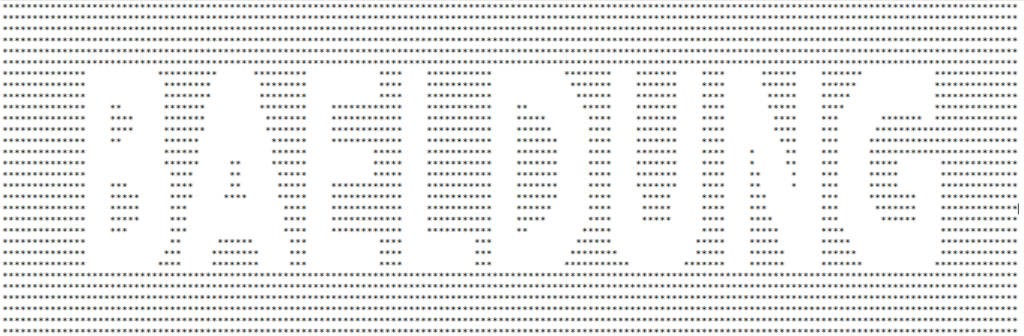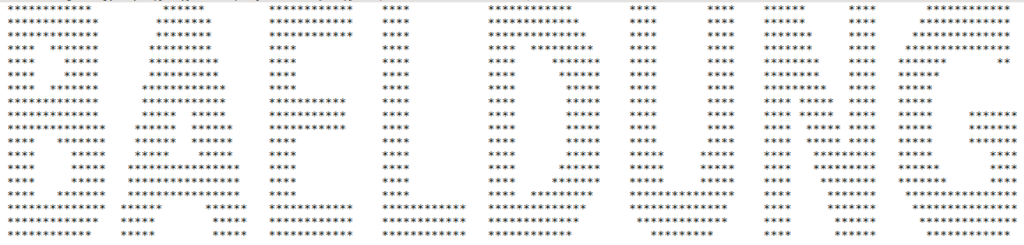## 1. Overview

In this article, we’ll discuss creating a graphical print of ASCII characters or Strings in Java, using concepts from the 2D graphics support of the language.

## 2. Drawing Strings With 2D Graphics

With the help of the Graphics2D class, it’s possible to draw a String as an image, achieved invoking the drawString() method.

Because Graphics2D is abstract, we can create an instance by extending it and implementing the various methods associated with the Graphics class.

While this is a tedious task, it’s often done by creating a BufferedImage instance in Java and retrieving its underlying Graphics instance from it:

``````BufferedImage bufferedImage = new BufferedImage(
width, height,
BufferedImage.TYPE_INT_RGB);
Graphics graphics = bufferedImage.getGraphics();``````

### 2.1. Replacing Image Matrix Indices With ASCII Character

When drawing Strings, the Graphics2D class uses a simple matrix-like technique where regions which carve out the designed Strings are assigned a particular value while others are given a zeroth value.

For us to be able to replace the carved area with desired ASCII character, we need to detect the values of the carved region as a single data point (e.g. integer) and not the RGB color values.

To have the image’s RGB color represented as an integer, we set the image type to integer mode:

``````BufferedImage bufferedImage = new BufferedImage(
width, height,
BufferedImage.TYPE_INT_RGB);``````

The fundamental idea is to replace the values assigned to non-zero indices of the image matrix with the desired artistic character.

While indices of the matrix representing the zero value will be assigned a single space character. The zero equivalent of the integer mode is -16777216.

## 3. ASCII Art Generator

Let’s consider a case where we need to make an ASCII art of the “BAELDUNG” string.

We begin by creating an empty image with desired width/height and the image type set to integer mode as mention in section 2.1.

To be able to use advanced rendering options of 2D graphics in Java, we cast our Graphics object to a Graphics2D instance. We then set the desired rendering parameters before invoking the drawString() method with the “BAELDUNG” String:

``````Graphics2D graphics2D = (Graphics2D) graphics;
graphics2D.setRenderingHint(RenderingHints.KEY_TEXT_ANTIALIASING,
RenderingHints.VALUE_TEXT_ANTIALIAS_ON);
graphics2D.drawString("BAELDUNG", 12, 24);``````

In the above, 12 and 24 represent respectively, the x and y coordinates for the point on the image where the text printing should start from.

Now, we have a 2D graphics whose underlying matrix contains two types of discriminated values; non-zero and zero indices.

But for us to get the concept, we will go through the 2-dimensional array (or matrix) and replace all values with the ASCII character “*” by:

``````for (int y = 0; y < settings.height; y++) {
StringBuilder stringBuilder = new StringBuilder();

for (int x = 0; x < settings.width; x++) {
stringBuilder.append("*");
}

if (stringBuilder.toString().trim().isEmpty()) {
continue;
}

System.out.println(stringBuilder);
}``````

The output of the above shows just a block of asterisks (*) as seen below:If we discriminate the replacement with “*” by replacing only the integer values equal to -16777216 with “*” and the rest with ” “:

``````for (int y = 0; y < settings.height; y++) {
StringBuilder stringBuilder = new StringBuilder();

for (int x = 0; x < settings.width; x++) {
stringBuilder.append(image.getRGB(x, y) == -16777216 ? "*" : " ");
}

if (stringBuilder.toString().trim().isEmpty()) {
continue;
}

System.out.println(stringBuilder);
}``````

We obtain a different ASCII art which corresponds to our string “BAELDUNG” but in an inverted carving like this:Finally, we invert the discrimination by replacing the integer values equal to -16777216 with ” ” and the rest with “*”:

``````for (int y = 0; y < settings.height; y++) {
StringBuilder stringBuilder = new StringBuilder();

for (int x = 0; x < settings.width; x++) {
stringBuilder.append(image.getRGB(x, y) == -16777216 ? " " : "*");
}

if (stringBuilder.toString().trim().isEmpty()) {
continue;
}

System.out.println(stringBuilder);
}``````

This gives us an ASCII art of the desired String:## 4. Conclusion

In this quick tutorial, we had a look at how to create ASCII art in Java using the inbuilt 2D graphics library.

While we have shown specifically for the text; “BAELDUNG”, the source code on Github provides a utility function that accepts any String.

Source code, as always, can be found over on GitHub.

### Get started with Spring and Spring Boot, through the Learn Spring course:

>> CHECK OUT THE COURSE